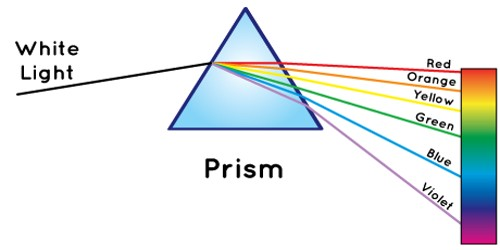# Prism definition in Refraction and Dispersion of light

Prism definition in Refraction and Dispersion of light

A transparent homogeneous medium bounded by three mutually intersecting plane surfaces is called a prism. Different physicists have different ideas about the definition of a prism. According to these ideas, any one of the following definitions can be given for a prism –

• A transparent homogeneous refracting medium bounded by two mutually inclined plane surfaces is called a prism.
• A transparent homogeneous retracting medium bounded by three rectangular and two triangular plane surfaces is called a prism. A prism has five planes.

So, if a transparent object is bounded by six rectangular surfaces in such a way as any two pairs of opposite surfaces are parallel and other two surfaces remain inclined to each other instead of remaining parallel, then it is called a prism.The surface through which light enters the prism and the surface through which light emerges are called refracting surfaces of the prism. The line along which the two refracting surfaces intersect is called the edge of the prism and angle between them is called the angle of a prism or refracting angle. The opposite side of the angle of the prism is called the base of the prism. A hypothetical plane perpendicular to the two refracting surfaces is called the section of the prism.

In figure AB and AC are refracting surfaces of a prism. ∠A is the angle of the prism, BC is the base of the prism, and ABC is the section of this prism.